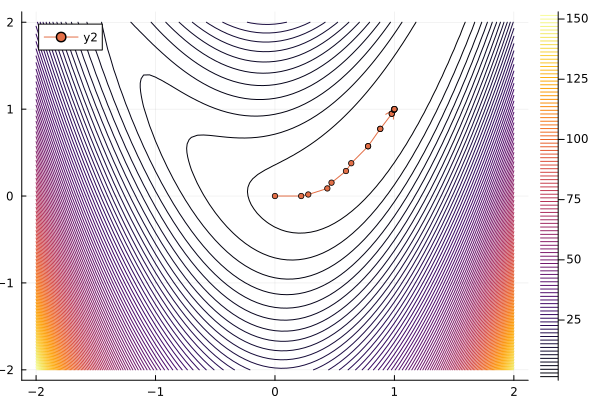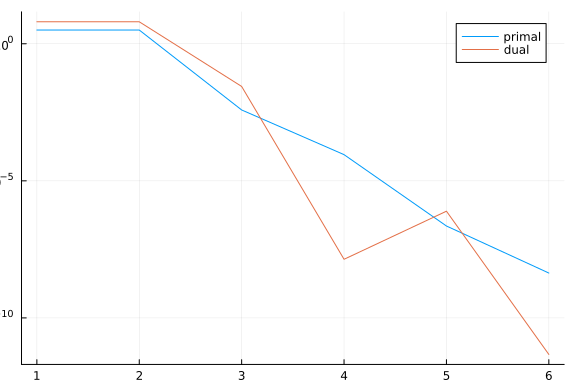# Using callbacksOne useful feature of our optimization solvers is the option to add a callback, i.e., a function that is called during the execution of the method, between iterations. It can be used for adding more logging information, for instance to plot the trace of the algorithm, or for having more control over the progress of the method, for instance by adding additional stopping criteria.

For these examples, let's consider a simple extension of the Rosenbrock function:

$\min\ \sum_{i=1}^N (x_{2i-1} - 1)^2 + (2i)^2 (x_{2i} - x_{2i-1}^2)^2$

We will implement this in JuMP and use NLPModelsJuMP to bridge the model to our NLPModels format.

using JuMP, NLPModelsJuMP

model = Model()
N = 10
@variable(model, x[1:2N])
@NLobjective(model, Min,
sum((x[2i - 1] - 1)^2 + (2i)^2 * (x[2i] - x[2i-1]^2)^2 for i = 1:N)
)

nlp = MathOptNLPModel(model)
NLPModelsJuMP.MathOptNLPModel
Problem name: Generic
All variables: ████████████████████ 20     All constraints: ⋅⋅⋅⋅⋅⋅⋅⋅⋅⋅⋅⋅⋅⋅⋅⋅⋅⋅⋅⋅ 0
free: ████████████████████ 20                free: ⋅⋅⋅⋅⋅⋅⋅⋅⋅⋅⋅⋅⋅⋅⋅⋅⋅⋅⋅⋅ 0
lower: ⋅⋅⋅⋅⋅⋅⋅⋅⋅⋅⋅⋅⋅⋅⋅⋅⋅⋅⋅⋅ 0                lower: ⋅⋅⋅⋅⋅⋅⋅⋅⋅⋅⋅⋅⋅⋅⋅⋅⋅⋅⋅⋅ 0
upper: ⋅⋅⋅⋅⋅⋅⋅⋅⋅⋅⋅⋅⋅⋅⋅⋅⋅⋅⋅⋅ 0                upper: ⋅⋅⋅⋅⋅⋅⋅⋅⋅⋅⋅⋅⋅⋅⋅⋅⋅⋅⋅⋅ 0
low/upp: ⋅⋅⋅⋅⋅⋅⋅⋅⋅⋅⋅⋅⋅⋅⋅⋅⋅⋅⋅⋅ 0              low/upp: ⋅⋅⋅⋅⋅⋅⋅⋅⋅⋅⋅⋅⋅⋅⋅⋅⋅⋅⋅⋅ 0
fixed: ⋅⋅⋅⋅⋅⋅⋅⋅⋅⋅⋅⋅⋅⋅⋅⋅⋅⋅⋅⋅ 0                fixed: ⋅⋅⋅⋅⋅⋅⋅⋅⋅⋅⋅⋅⋅⋅⋅⋅⋅⋅⋅⋅ 0
infeas: ⋅⋅⋅⋅⋅⋅⋅⋅⋅⋅⋅⋅⋅⋅⋅⋅⋅⋅⋅⋅ 0               infeas: ⋅⋅⋅⋅⋅⋅⋅⋅⋅⋅⋅⋅⋅⋅⋅⋅⋅⋅⋅⋅ 0
nnzh: ( 85.71% sparsity)   30              linear: ⋅⋅⋅⋅⋅⋅⋅⋅⋅⋅⋅⋅⋅⋅⋅⋅⋅⋅⋅⋅ 0
nonlinear: ⋅⋅⋅⋅⋅⋅⋅⋅⋅⋅⋅⋅⋅⋅⋅⋅⋅⋅⋅⋅ 0
nnzj: (------% sparsity)

Counters:
obj: ⋅⋅⋅⋅⋅⋅⋅⋅⋅⋅⋅⋅⋅⋅⋅⋅⋅⋅⋅⋅ 0                 grad: ⋅⋅⋅⋅⋅⋅⋅⋅⋅⋅⋅⋅⋅⋅⋅⋅⋅⋅⋅⋅ 0                 cons: ⋅⋅⋅⋅⋅⋅⋅⋅⋅⋅⋅⋅⋅⋅⋅⋅⋅⋅⋅⋅ 0
cons_lin: ⋅⋅⋅⋅⋅⋅⋅⋅⋅⋅⋅⋅⋅⋅⋅⋅⋅⋅⋅⋅ 0             cons_nln: ⋅⋅⋅⋅⋅⋅⋅⋅⋅⋅⋅⋅⋅⋅⋅⋅⋅⋅⋅⋅ 0                 jcon: ⋅⋅⋅⋅⋅⋅⋅⋅⋅⋅⋅⋅⋅⋅⋅⋅⋅⋅⋅⋅ 0
jgrad: ⋅⋅⋅⋅⋅⋅⋅⋅⋅⋅⋅⋅⋅⋅⋅⋅⋅⋅⋅⋅ 0                  jac: ⋅⋅⋅⋅⋅⋅⋅⋅⋅⋅⋅⋅⋅⋅⋅⋅⋅⋅⋅⋅ 0              jac_lin: ⋅⋅⋅⋅⋅⋅⋅⋅⋅⋅⋅⋅⋅⋅⋅⋅⋅⋅⋅⋅ 0
jac_nln: ⋅⋅⋅⋅⋅⋅⋅⋅⋅⋅⋅⋅⋅⋅⋅⋅⋅⋅⋅⋅ 0                jprod: ⋅⋅⋅⋅⋅⋅⋅⋅⋅⋅⋅⋅⋅⋅⋅⋅⋅⋅⋅⋅ 0            jprod_lin: ⋅⋅⋅⋅⋅⋅⋅⋅⋅⋅⋅⋅⋅⋅⋅⋅⋅⋅⋅⋅ 0
jprod_nln: ⋅⋅⋅⋅⋅⋅⋅⋅⋅⋅⋅⋅⋅⋅⋅⋅⋅⋅⋅⋅ 0               jtprod: ⋅⋅⋅⋅⋅⋅⋅⋅⋅⋅⋅⋅⋅⋅⋅⋅⋅⋅⋅⋅ 0           jtprod_lin: ⋅⋅⋅⋅⋅⋅⋅⋅⋅⋅⋅⋅⋅⋅⋅⋅⋅⋅⋅⋅ 0
jtprod_nln: ⋅⋅⋅⋅⋅⋅⋅⋅⋅⋅⋅⋅⋅⋅⋅⋅⋅⋅⋅⋅ 0                 hess: ⋅⋅⋅⋅⋅⋅⋅⋅⋅⋅⋅⋅⋅⋅⋅⋅⋅⋅⋅⋅ 0                hprod: ⋅⋅⋅⋅⋅⋅⋅⋅⋅⋅⋅⋅⋅⋅⋅⋅⋅⋅⋅⋅ 0
jhess: ⋅⋅⋅⋅⋅⋅⋅⋅⋅⋅⋅⋅⋅⋅⋅⋅⋅⋅⋅⋅ 0               jhprod: ⋅⋅⋅⋅⋅⋅⋅⋅⋅⋅⋅⋅⋅⋅⋅⋅⋅⋅⋅⋅ 0

To solve this problem, we can use trunk from JSOSolvers.jl.

using JSOSolvers

output = trunk(nlp)
println(output)
Generic Execution stats
status: first-order stationary
objective value: 8.970912001856092e-17
primal feasibility: 0.0
dual feasibility: 8.338702542070883e-9
solution: [0.9999999917126897  0.9999999825882647  0.9999999997312826  0.999999999455177 ⋯ 1.0000000026501263]
iterations: 18
elapsed time: 1.0835239887237549

The callback function is any function with three input arguments: nlp, solver and stats. nlp is the exact problem we are solving, solver is a solver structure that keeps relevant values, and stats is the output structure, which keeps updated values every iteration. Look at the help of the solver for more information on which values are available.

A few of the most important values are:

• solver.x: The current iterate

• solver.gx: The current gradient

• stats.objective: The objective value at the current iterate

• stats.dual_feas: The dual feasibility value

• stats.primal_feas: The primal feasibility value

• stats.iter: The number of iterations

Using these, we can, for instance, create a trace of the method in the first two variables:

using NLPModels, Plots

X = zeros(0, 2)
cb = (nlp, solver, stats) -> begin
global X = [X; solver.x[1:2]']
end

output = trunk(nlp, callback=cb)

contour(-2:0.05:2, -2:0.05:2,
(x1, x2) -> obj(nlp, [x1; x2; output.solution[3:end]]),
levels = 100,
)
plot!(X[:,1], X[:,2], l=:arrow, m=(3))Here, NLPModels is used to access the function obj.

We can also use callbacks to exert more control over the algorithm. For instance, to implement a check on whether the objective value is stalling, we can define the following callback:

fold = -Inf
cb = (nlp, solver, stats) -> begin
global fold
f = stats.objective
if abs(f - fold) / (abs(f) + abs(fold) + 1e-8) < 1e-3
stats.status = :user
end
fold = f
end

output = trunk(nlp, callback=cb, rtol=0.0, atol=0.0)
println(output)
Generic Execution stats
status: user-requested stop
objective value: 1.405115328770847e-20
primal feasibility: 0.0
dual feasibility: 1.4364101353965366e-10
solution: [0.9999999999573873  0.9999999999104003  0.999999999934689  0.9999999998670257 ⋯ 0.999999999938523]
iterations: 18
elapsed time: 0.005786895751953125

Above, we set the status of the algorithm to :user, so that it stops with a user-request stop flag.

trunk is not the only solver that accepts callbacks. All solvers in JSOSolvers, as well as percival and dci from Percival.jl and DCISolver.jl do as well. Here is an example:

using Percival

@NLconstraint(model, [i=1:N], x[2i]^2 + x[2i-1]^2 <= 1.0)
nlp = MathOptNLPModel(model)

primal_history = Float64[]
dual_history = Float64[]
cb = (nlp, solver, output) -> begin
push!(primal_history, output.primal_feas)
push!(dual_history, output.dual_feas)
end
output = percival(nlp, callback=cb)

plt = plot()
plot!(plt, primal_history, lab="primal", yaxis=:log)
plot!(plt, dual_history, lab="dual")